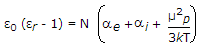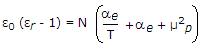# Materials and Components – Section 3(Page 2)

Materials and Components – Section 3

11.The resistivity of intrinsic semiconductors, at room temperature, is of the order of
 A. 1 Ω-m B. 1000 Ω-m C. 106 Ω-m D. 10-3 Ω-m

12.Assertion (A): Ferromagnetic materials possess ferromagnetic properties only when temperature is less than paramagnetic curie temperature.

Reason (R): For a ferromagnetic materialfor T > θf.
 A. Both A and R are true and R is correct explanation of A B. Both A and R are true but R is not correct explanation of A C. A is true but R is false D. A is false but R is true

13. In terms of classical representation of electrons
 A. an atom is essentially empty B. an atom is essentially full C. an atom may be essentially empty or full depending on the material D. an atom may be essentially empty or full depending on temperature

14. To produce magnetic flux, mmf is necessary.
 A. True B. False

15. For a polyatomic gas, the equation relating dielectric constant ∈r with molecular properties is
 A.B.C.D.16. The relaxation time of electrons in a metal is of the order of
 A. 10-6 secs B. 10-10 secs C. 10-14 secs D. 10-20 secs

17. Assertion (A): For elemental dielectrics, P = NaeEi where N is number of atoms/m3, P is polarization, ae is electronic polarization and Ei is internal electric field.

Reason (R): In elemental dielectrics there are no permanent dipoles or ions.

 A. Both A and R are true and R is correct explanation of A B. Both A and R are true but R is not correct explanation of A C. A is true but R is false D. A is false but R is true

Explanation:

In elemental dielectrics Pi = Po = 0.

18. The circumference of an electron orbit is equal to integer times the wavelength of electron.
 A. True B. False

19. In a specimen of silicon steel, a field strength of 200 A/m causes a flux density of 0.5 T. In a specimen of cast iron, the same H would cause B to be
 A. 0.5 T B. less than 0.5 T C. more than 0.5 T D. 0.5 T or more or less